# Area And Perimeter Of Triangle - Definition with Examples

The Complete K-5 Math Learning Program Built for Your Child

• 40 Million Kids

Loved by kids and parent worldwide

• 50,000 Schools

Trusted by teachers across schools

• Comprehensive Curriculum

Aligned to Common Core

## Area and Perimeter of Triangle

Perimeter of a Triangle

The perimeter of a polygon is the total length of the boundary. For a triangle, we can find it by adding the lengths of its three sides.

We can find the length of the fencing required for a triangular park by finding the perimeter of the triangle.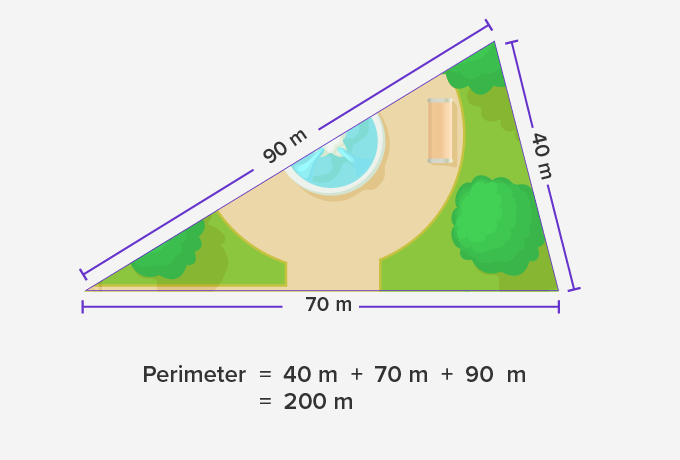A serving tray as shown forms an equilateral triangle – that is, a triangle with three equal sides. Let each side be 20 cm long.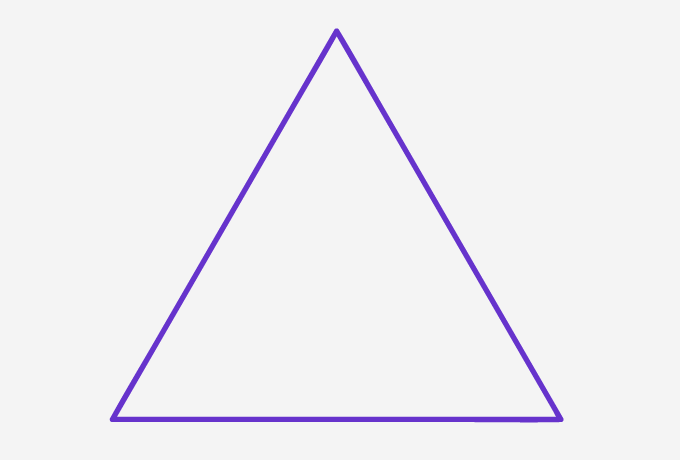To find the total length of a decorative lace to be pasted on outside borders, one needs to find the perimeter of the triangle. Since all the three sides of the triangle are of equal length, we can find the perimeter by multiplying the length of each side by 3.

20 + 20 + 20 = 3 × 20 = 60 cm.

Thus, the perimeter of an equilateral triangle is 3 times the length of each side.

Area of a triangle

Area of a two-dimensional shape is the space occupied by the shape. This area can be found by dividing the shape into unit squares and determining the number of unit squares in the shape as each unit square occupies one square unit space.

Consider a rectangle of length 4 cm and width 3 cm. It can be filled with 3 rows and 4 columns of unit squares and therefore the area is 3 times 4 or 12 square centimeter. That is, the area of a rectangle is the product of its length and width.

A rectangle can be divided into two congruent triangles.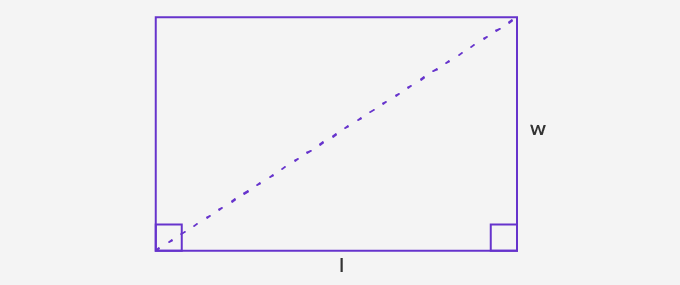So, the area of each triangle is half the area of the rectangle. That is 1x l x w.

Consider a scalene triangle △ABC. Note that, to write the area of a triangle as half the area of a rectangle, we need to have the height perpendicular to the base. So, draw a perpendicular from a vertex to the opposite side.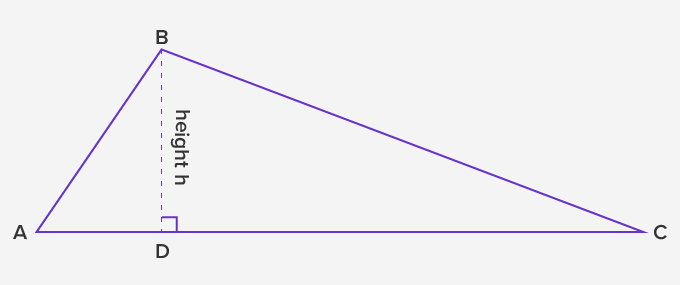Here, BD is the perpendicular drawn from the vertex B to the opposite side AC.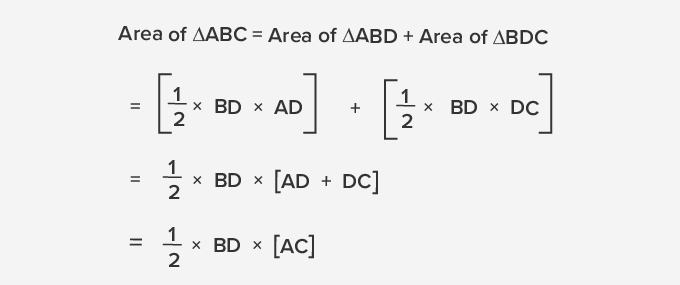Thus, the area of a triangle is half the product of its base and height.

 Fun Facts The area A of an equilateral triangle of side length s cm can be calculated using the formula A = √3⁄4 s2. The value of √3 is about 1.73. Thus, the approximate area becomes, A = 0.4325 s2.

Won Numerous Awards & Honors